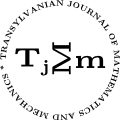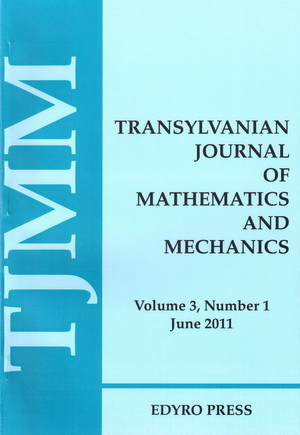ISSN: 2067-239X
ISSN(on-line): 2067-239X

Indexed in:
Mathematical Reviews
Zentralblatt MATH
EBSCO

Front cover# Volume 6 (2014), Number 1

## A Differential Sandwich-type Result Using an Extended Generalized Sălăgean Operator and Extended Ruscheweyh Operator

### Author(s): LORIANA ANDREI

Abstract: The purpose of this paper is to introduce sufficient conditions for strong differential subordination and superordination involving the extended derivative operator $D{R}_{\lambda }^{m,n}$ and also to obtain sandwich-type result.

## Mathematical Models Regarding the Basic Informative Elements for the Classical Computers and the Quantum Computers Respectively

### Author(s): MALVINA BAICA and MIRCEA CARDU

Abstract: In this paper we use the results obtained in a previous paper  regarding the pairs of the DUAL roots (+1 OR -1) and respectively BIPOLAR roots (+1 AND -1) of a couple of equations “seen in the mirror” (${y}^{2}±1=0$) and with the help of a mathematical artifice we arrive to the basic informative elements characteristic to the Classical Computers (1 OR 0) and respectively to the Quantum Computers (1 AND 0). In this way we also prove that the two categories of the basic informative elements have a joint origin in the couple of the equations mentioned above.

## Starlikeness of Libera Operator on Certain Concave Univalent Functions

### Author(s): IBTISAM AL-DAWISH and MASLINA DARUS

Abstract: Let ${C}_{0}\left(\alpha \right)$ denote the class of concave univalent functions deﬁned in the open unit disk $𝔻$. Each function $f\in {C}_{0}\left(\alpha \right)$ maps the unit disk $𝔻$ onto the complement of an unbounded convex set. In this paper, we shall prove that the Libera operator $F\left(z\right)=\frac{2}{z}{\int }_{0}^{z}f\left(t\right)dt$ is starlike by ﬁnding certain conditions on $Re\phantom{\rule{0.3em}{0ex}}2{f}^{\prime }$.

## A New Approach to One-Dimensional Oscillators in Relativistic Quantum Mechanics

### Author(s): LAUREAN HOMORODEAN

Abstract: A new approach to the one-dimensional oscillatory motion of a relativistic quantum particle with the spin $\frac{1}{2}$ is presented. It is based on a modiﬁed form of the Hamilton operator of the particle. As particular cases, the one-dimensional Dirac oscillator and the one-dimensional oscillator with equidistant energy levels are discussed. In this context, the ﬁrst oscillator appears as an ancient representative, while the second oscillator is a new representative of an entire class of relativistic quantum one-dimensional oscillators.

## On the Maximum Term and Lower Order of Entire Monogenic Functions

### Author(s): SUSHEEL KUMAR and G.S. SRIVASTAVA

Abstract: In the present paper, we study the growth properties of entire monogenic functions. The characterizations of lower order of entire monogenic functions have been obtained in terms of their Taylor’s series coeﬃcients. Also we have obtained some inequalities between order, type, maximum term and central index of entire monogenic functions.

## A Note to Geometry of Cosserat Media and Deformation Bundles

### Author(s): MIROSLAV KUREŠ

Abstract: We study Cosserat media from the geometric point of view; in particular, we present a construction of Cosserat deformation bundles and demonstrate the role of the velocities bundles.

## On the Convergence of Modiﬁed Noor Iteration Method for Nearly Lipschitzian Mappings in Arbitrary Real Banach Spaces

Abstract: In this present paper, we employed a modiﬁed Noor iteration method introduced by Raﬁq . Some strong convergence theorems of this iteration scheme are established for three nearly uniformly Lipschitzian mappings if at least one of these maps is uniformly Lipschitzian mapping. Our results extend and improve the recent ones proved by Chang et al., Kim et al., Olaleru and Mogbademu, Ofoedu and many others.

## State Space Approach for Solving Transient Queuing Systems

### Author(s): T.S.L. RADHIKA and B.L.R. DEEPIKA

Abstract: In the present paper, we consider a transient queuing system and ﬁnd its solution using the state space approach. For this, the given interval of study [0,T] is sub divided into small sub intervals, where it is reasonable to assume that the instantaneous arrival and service rates are constants. The system of equations together with the initial conditions is solved for an analytical expression for ${P}_{n}$s’ in each interval. The solution obtained at the right end point of the previous sub interval is taken as the initial condition in the sub sequent interval. The developed method is illustrated for an (M/M/1):(FCFS/m/∞) system.

## On the Natural ${q}^{2}$-analogue of the Generalized Gegenbauer Form

### Author(s): I. BEN SALAH and L. KHÉRIJI

Abstract: The aim of this paper is to highlight a ${q}^{2}$-analogue of the generalized Gegenbauer polynomials orthogonal with respect to the form $\mathsc{𝒢}\left(\alpha ,\beta ,{q}^{2}\right)$. Integral representation and discrete measure of $\mathsc{𝒢}\left(\alpha ,\beta ,{q}^{2}\right)$ are given for some values of parameters.